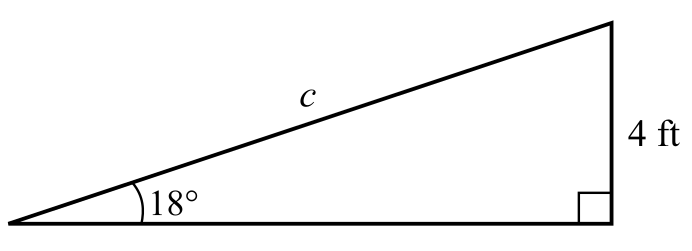Chapter 8.2, Problem 74E### Calculus: An Applied Approach (Min...

10th Edition
Ron Larson
ISBN: 9781305860919

#### Solutions

Chapter
Section### Calculus: An Applied Approach (Min...

10th Edition
Ron Larson
ISBN: 9781305860919
Textbook Problem
1 views

# Skateboard Ramp A skateboard ramp with a height of 4 feet has an angle of elevation of 18° (see figure). What is the length c of the skateboard ramp?To determine

To calculate: The length c of the skateboard ramp when the angle of elevation is 18° and height of ramp is 4 feet.Explanation

Given Information:

The figure is shown below,

Formula used:

Definition of sine function:

sinθ=yr

Where, x is the length of the side adjacent to θ, y is the length of the side opposite to θ, and r=x2+y2 is the length of hypotenuse which is not equal to 0.

Calculation:

Consider the figure,

Now apply sine on triangle ABC,

sinθ=ABAC

Substitute the value of θ=18°,

### Still sussing out bartleby?

Check out a sample textbook solution.

See a sample solution

#### The Solution to Your Study Problems

Bartleby provides explanations to thousands of textbook problems written by our experts, many with advanced degrees!

Get Started

#### In Exercises 516, evaluate the given quantity. log218

Finite Mathematics and Applied Calculus (MindTap Course List)

#### 1. Solve the following for x. (a) (b) (c)

Mathematical Applications for the Management, Life, and Social Sciences

#### True or False: is conservative.

Study Guide for Stewart's Multivariable Calculus, 8th